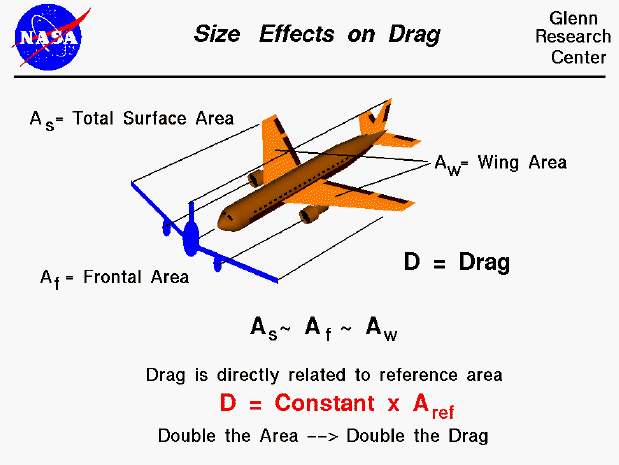The amount of drag generated depends on the size of the object. Drag is an aerodynamic force and therefore depends on the pressure variation of the air around the body as it moves through the air. The total aerodynamic force is equal to the pressure times the surface area around the body; drag is the component of this force along the flight direction. Like the other aerodynamic force, lift, the drag is proportional to the area of the object. Doubling the area will double the drag.

Unlike lift, however, there are several different areas from which to choose when developing the reference area used in the drag equation. If we think of drag as being caused by friction between the air and the body, a logical choice would be the total surface area (As) of the body. If we think of drag as being a resistance to the flow, a more logical choice would be the frontal area (Af) of the body which is perpendicular to the flow direction. This is the area shown in blue on the figure. Finally, if we want to compare with the lift coefficient, we should use the same area used to derive the lift coefficient, the wing area, (Aw). Each of the various areas are proportional to the other areas, as designated by the "~" sign on the figure. Since the drag coefficient is determined experimentally, by measuring the drag and measuring the area and performing the necessary math to produce the coefficient, we are free to use any area which can be easily measured. If we choose the wing area, the computed coefficient will have a different value than if we had chosen the cross-sectional area, but the drag is the same, and the coefficients are related by the ratio of the areas. In practice, drag coefficients are reported based on a wide variety of object areas. In the report, the aerodynamicist must specify what area is used and when using the data the reader may have to convert the drag coefficient using the ratio of the areas.

Guided Tours